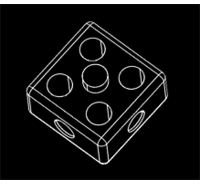Applications of the CAD Software – What is Geometric Modeling?

What is Geometric Modeling?

There are number of applications of the CAD software, one of the most popular applications being geometric modeling. First of all let us see what is geometric modeling? The computer compatible mathematical description of the geometry of the object is called as geometric modeling. The CAD software allows the mathematical description of the object to be displayed as the image on the monitor of the computer.

Steps for Creating the Geometric Model

There are three steps in which the designer can create geometric models by using CAD software, these are:

1) Creation of basic geometric objects: In the first step the designer creates basic geometric elements by using commands like points, lines, and circles.

2) Transformations of the elements: In the second step the designer uses commands like achieve scaling, rotation and other related transformations of the geometric elements.

3) Creation of the geometric model: During the final step the designer uses various commands to that cause integration of the objects or elements of the geometric model to form the desired shape.

During the process of geometric modeling the computer converts various commands given from within the CAD software into mathematical models, stores them as the files and finally displays them as the image. The geometric models created by the designer can open at any time for reviewing, editing or analysis.

Representation of the Geometric Models

Of the various forms of representing the objects in geometric models, the most basic is wire frames. In this form the object is displayed by interconnected lines as shown in the figure below (source: Wikipedia). There are three types of wire frame geometric modeling, these are: 2D, 2.1/2D and 3D. They have been described below:

1) 2D: It stands of two dimensional view and is useful for flat objects.

2) 2.1/2D: It gives views beyond the 2D view and permits viewing of 3D object that has no sidewall details.

3) 3D: The three dimension representation allows complete three-dimensional viewing of the model with highly complex geometry. Solid modeling is the most advanced method of geometric modeling in three dimensions.Reference

Book: CAD/CAM: Computer Aided Design and Manufacturing by Mikell P. Groover and Emory W. Zimmers

This post is part of the series: Applications of CAD

This is the series of articles describing various applications of CAD software like geometric modeling, engineering analysis, FEA, design review and evaluation, drafting etc.